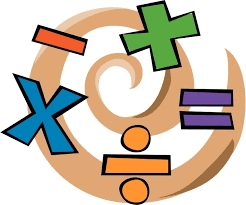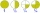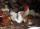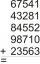# Complicated sum minus product

What must be subtracted from the sum of 3/8 and 5/16 to get difference equal to the product of 5/8 and 3/16?

x =  0.5703

### Step-by-step explanation:Did you find an error or inaccuracy? Feel free to write us. Thank you!Tips to related online calculators
Need help to calculate sum, simplify or multiply fractions? Try our fraction calculator.

## Related math problems and questions:

• Product of the sum and differenceCalculate the product of the sum and difference of numbers -7 and -2.
• Quotient 3If the quotient of 8/13 and 2 is subtracted from the product of 1 3/4 and 8/21, what is the difference?
• SixteenSixteen minus the quotient of 12 and what number is thirteen?
• Quotient and productIf the quotient of [8/5 divided by 8/10] is added to the product of [8/14 x 7/12 x 3/8], what is the sum?
• Evaluate fractionsThe difference  of 5 1/2 and 2/3 is added to the product of 5/6 and 1/2To three-eighths of one third, we add five quarters of one half and multiply the sum by four. How much will we get?
• When 5When 5 is subtracted from both my numerator and denominator I become 3/4. What fraction i am?
• Sum and productThe sum of the real numbers x and y is 12. Their difference is 8. What is the value of xy?
• Product increasedWhen the product of 2/3 and 6/10 is increased by 2/5, the result is?
• Hens11 hens will eat spilled grain from 6AM to 16 PM. At 11 hour grandmother brought 4 hens from the neighbors. At what time was grain out?
• Two numbers and its productThe product of two numbers are 2/3. If on of them is 1/10, what is the other?Fill letters instead of digits so the indicated sum (equal letters represent equal digits). What number is hidden under the letter J? A A H A H O A H O J -------------------------- 4 3 2 1The sum of 3 mixed numbers is 20 13/15. two of the numbers are 6 1/3 and 7 5/6. what is the third number?Which decimals when subtracted equal 3.89: a - b = 3.89Bill is cutting boards to build a bookshelf for his apartment. While cutting boards he accidently cut 3/16th ft. His board is 3 3/4th, how long should it have been?Find the area and the perimeter of a rectangle of length 45 1/2 cm and breadth 16 2/3 cm.How many three-tenths are there in two and one-fourths?